# CRACK IBPS Clerk Prelims – Direction test Day 24

Q.1) Gopal ranks sixteenth from the top and eighteenth from the bottom. How many students are there in a class?

a) 31

b) 32

c) 33

d) 34

e) None of these

(c)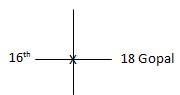=> 16 + 18 – 1 = 33

Q.2) Five person A, B, C, D and E are standing in a line according to their heights. C is taller than D and E. B is shorter than only one person. Then who is the tallest person standing in a line?

a) A

b) C

c) D

d) Cannot be determined

e) None of these

(d)

A > B > C > D/E

Q.3) In a class of 50 students, Jayanthi ranks thirteenth from the top, then what is her rank from the bottom?

a) 37

b) 38

c) 36

d) Cannot be determined

e) None of these

(b)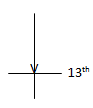50 – 13 + 1 => 38

Q.4) In a row, Mani is 15th from the left end and Rajiv is 9th from the right end. What is Rajiv’s rank from the left end of the row, if there are 4 students’ sits between Mani and Rajiv?

a) 19

b) 28

c) Cannot be determined

d) 27

e) None of these

(c)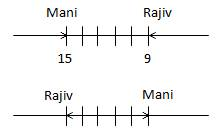Q.5) In a row of 35 boys, Karthi is fourth to the right of kunal. If kunal is 19th

from the right end, then what is karthi’s position from the left end?

a) 21

b) 20

c) 26

e) None of these

(a)

35 – 15 + 1 = 21

Q.6) In a row of boys, Kevin is 20th from the right end and Daniel is 15th from the left end. When Kevin and Daniel interchange their positions, Kevin will be 25th from the right end. Which of the following will be Daniel’s position from the left end (after interchanging)?

a) 18

b) 19

c) 20

d) 21

e) None of these

(c)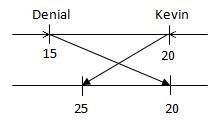Q.7) In a row of boys, Sundar is 11th from the left end and Mohan is 31st from the right end. Karthi is 15th to the right of Sundar and 4th to the left of Mohan. How many boys are there in the row?

a) 61

b) 60

c) 59

d) Cannot be determined

e) None of these

(b)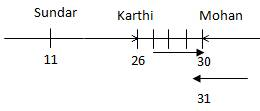30 + 31 – 1 = 60

D.8-10) Study the following information and answer the questions given below:

Among five friends Kavitha, Raju, Selva, Neeraj and divya scored different marks. Neeraj scored more marks than only Divya and Raju. Selva scored more than Neeraj, but not scored highest mark. The one who scored second highest got 85 mark.

Q.8) If Divya got the lowest mark, then who got the second last mark?

a) Neeraj

b) Selva

c) Raju

d) Kavitha

e) None of these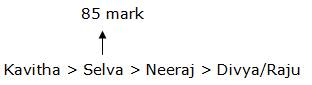Q.9) If the average score of Selva and Divya is 60, then Divya got how much mark?

a) 30

b) 32

c) 85

d) 35

e) None of theseAverage score of Selva and Divya is 60

Total score of Selva and Divya is 120

Selva = 85

Divya = 120 – 85 = 35

Q.10) If Divya scored more than only one person and got 75 marks, then Neeraj scored how much marks?

a) 67

b) 90

c) 87

d) 82

e) None of these